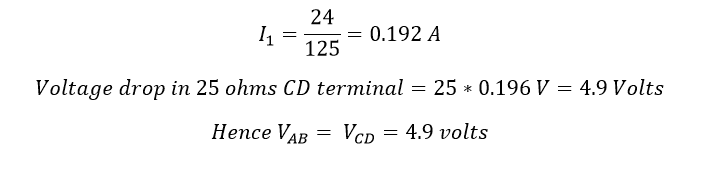# What is Open Circuit ?

## Open circuit:

The circuit is said to be open if there is a gap, or break or any interruption in a circuit’s current flowing path.

In the below-mentioned circuit, A and B are two terminals in open condition, here the current flow to the load circuit (resistor) is zero. Here V is applied Voltage across the terminal, I is the circulating voltage and R is the resistive load or instead of resistive load, we can connect inductive load or others. Let us calculate the resistance between the open circuit.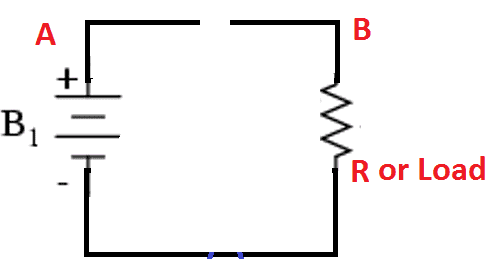According to Ohm’s law.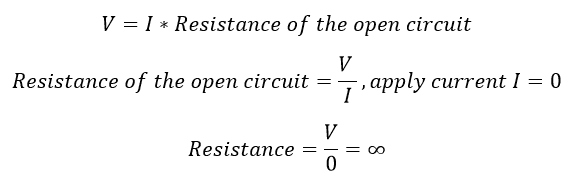An open circuit has infinite resistance, the voltage across the open-circuited terminal AB is known as open circuit voltage.

## Key Points in open circuit:

• In an open circuit I=0
• The resistance of an open circuit R= infinity
• The voltage of an open circuit is maximum (refer the problem 3)

Example for the open circuit: Switches, contactor, circuit breaker etc.

Note: Do not confuse the term between open circuit and short circuit.

Learn More:   7 Purpose of NGR Neutral Grounding Resistor Transformer & Generator

## The problem in open circuit:

### Problem: 1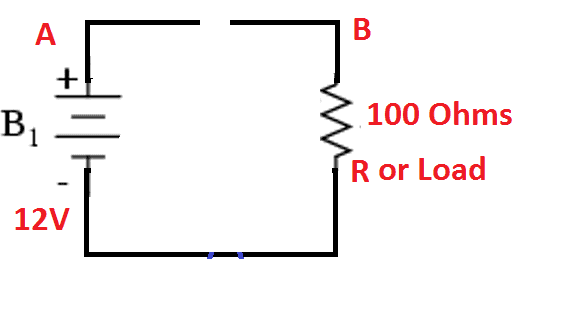Find the voltage across the terminal AB for given circuit. A 100 ohms resistor is connected in series with the battery 12 V.

Solution:

Since the terminal AB is open in the circuit, here the current flow is equal to zero. There is zero voltage drop across the load resistor, apply Kirchhoff voltage rule… then we get the formula…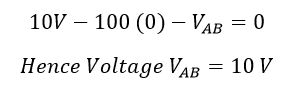### Problem: 2

Find the voltage across the terminal C D…

Solution:

The current flowing in the network is zero, apply Kirchhoff voltage rule to get the voltage across the terminal CD.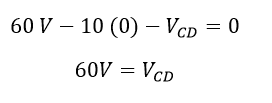## Problem 3:

Find the voltage across AB terminal: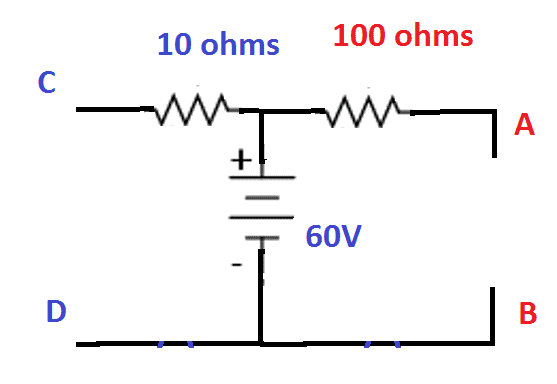Solution:

This problem is the little bit different from above two, here the terminals A and B are open, current flow through the 10-ohm resistor is zero, hence the voltage drop across the resistor is zero. Therefore, the voltage drop across the terminal AB is equal to the voltage drop in 25-ohm resistor CD terminal…. Apply ohms law because of the current I3=0 due to open terminal AB…